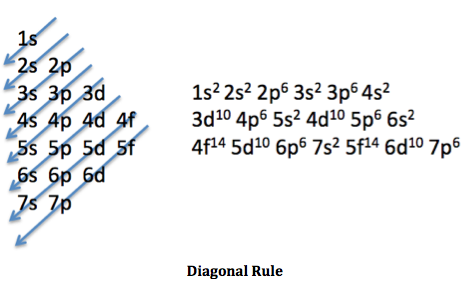# Chemistry I - Polyatomic Ions, Electron Configurations, Bonding Models,

20 Questions | Total Attempts: 167SettingsThis is a quiz on certain topics in a Chemistry I class. The 3 topics covered are: 1) Polyatomic ions: Do you know you polyatomic ions, their charges, how to form a bond? 2) Electron Configurations: Are s,p,d,f familiar? 3) Bonding Models: Ionic, Molecular Covalent, Metallic, Network Covalent Note: You ARE allowed to use the periodic table at anytime throughout the quiz.

• 1.
What is the charge of the anion: CO3 ?
• A.

1-

• B.

2-

• C.

-

• D.

0

• E.

+

• 2.
Determine the correct chemical name for: Fe(NO3)2
• A.

Iron (II) Nitride

• B.

Iron (I) Nitrate

• C.

Iron (II) Nitride

• D.

Iron (II) Nitrate

• E.

Iron (I) Nitride

• 3.
Determine the correct chemical formula for: Sodium Phosphate
• A.

Na5PO3

• B.

NaPO2

• C.

Na4PO3

• D.

Na2PO

• E.

Na3PO4

• 4.
What is the same between Manganese (II) Chloride and Manganese (IV) Chloride?
• A.

Charge of Manganese

• B.

Charge of Chlorine

• C.

Overall charge

• D.

2 of the above

• E.

All of the above

• 5.
Determine the number of copper in one molecule of: Copper (I) Sulfate
• A.

0

• B.

1

• C.

2

• D.

3

• E.

4

• 6.
Which of the following is the correct electron configuration for Carbon?
• A.

1s^2 2s^2 2s^3

• B.

2s^2 2p^2 2p^2

• C.

1s^2 2s^2 2p^2

• D.

2s^1 2s^2 2p^2

• E.

1s^2 1p^2 2p^2

• 7.
The maximum number of electrons in the 3d subshell is:
• A.

2

• B.

6

• C.

10

• D.

14

• 8.
Elements with 1-9 electrons in their 4d subshell are located:
• A.

Alkaline Earth Metals

• B.

Halogens

• C.

Period 4 Transition metals

• D.

Period 5 Transition metals

• E.

Noble Gases

• 9.
Elements with 5 electrons on their outermost p shell are located:
• A.

Alkaline Earth Metals

• B.

Halogens

• C.

Period 5 Transition metals

• D.

Period 4 Transition metals

• E.

Noble Gases

• 10.
The electron configuration: 1s^2 2s^2 2p^6 3s^2 3p^6 4s^2 3d^6   corresponds to the element:
• A.

Iron, Fe

• B.

Argon, Ar

• C.

Tin, Sn

• D.

None of the above

• E.

The above electron configuration is incorrect. It should be 1s^2 2s^2 2p^6 3s^2 3p^6 3d^6 4s^2

• 11.
The maximum number of electrons in the f subshell would correspond with:
• A.

The number of elements in the transition metals

• B.

The number of elements in the lanthanoids and actinoids

• C.

The number of periods on the periodic table

• D.

The number of groups on the periodic table

• E.

The number of protons in the atom

• 12.
Which bonding model best describes: CH4 (g) ? (Note: CH4 is a gas)
• A.

Ionic

• B.

Network Covalent

• C.

Metallic

• D.

Molecular Covalent

• E.

Structural Covalent

• 13.
Which bonding model best describes: SiO2 (s) ? (Note: SiO2 (s) is very hard)
• A.

Ionic

• B.

Network Covalent

• C.

Metallic

• D.

Molecular Covalent

• E.

Structural Covalent

• 14.
Which of the following is NOT a property of Ionic substances?
• A.

They are made up a cation and anion

• B.

They conduct electricity when dry

• C.

They dissolve in water

• D.

They conduct electricity when dissolved

• E.

None of the above

• 15.
Which of the following is NOT a property of Metallic substances?
• A.

Dissolves in water

• B.

Conducts electricity

• C.

Some are hard solids

• D.

• E.

None of the above

• 16.
Which of the following is NOT a property of Molecular Covalent substances?
• A.

They are made entirely of non-metal atoms

• B.

They always dissolve in water

• C.

They don't conduct electricity

• D.

They can be liquids or gasses

• E.

None of the above

• 17.
Which of the following is NOT a property of Network Covalent substances?
• A.

They don't dissolve

• B.

They don't conduct electricity

• C.

They are extremely hard

• D.

They are made up of non-metal and metalloid atoms

• E.

None of the above

• 18.
Predict the bonding model for KI, potassium iodine
• A.

Ionic

• B.

Molecular Covalent

• C.

Metallic

• D.

Network Covalent

• E.

It cannot be determined

• 19.
CO2 (Carbon dioxide) is molecular covalent. Which piece of evidence best supports this?
• A.

Carbon dioxide dissolves in water

• B.

It does not conduct electricity

• C.

It is a gas at room temperature

• D.

It is made up of non-metal atoms

• E.

It is polyatomic

• 20.
Diamond (C) is a very hard substance. Which bonding model best describes it?
• A.

Ionic

• B.

Molecular Covalent

• C.

Metallic

• D.

Network Covalent

• E.

Structural Covalent

Related TopicsBack to top